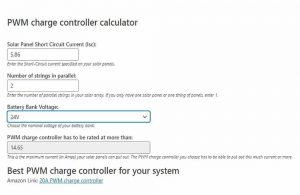# PWM calculator: How to easily choose the right PWM charge controller for your system

The PWM calculator below will tell you which PWM is best for your system based on the maximum current your solar panels can put out.

All you have to do is enter a few details that describe your solar system and the calculator will display a link to a suitable PWM charge controller.

## PWM calculator

Solar panel short-circuit current (Isc): In this field, you should enter the Isc specified on your solar panels. You can find this value on the specification label on your solar panel or by googling the model.

Number of strings in parallel: In this field, please enter the number of parallel strings. For example, if you have 3 panels all connected in parallel (positive to positive and negative to negative), enter 3.

Battery bank voltage: In this field enter the nominal voltage of your battery bank. This value will not influence the rating of the PWM charge controller you need, but it will help the calculator find the best PWM deal.

If you don’t really understand the values you’re supposed to enter or if you’re just more interested in the how I recommend reading the next section.

## How to choose the right PWM charge controller for your PV system

To select the right PWM solar charge controller, you’ll need to calculate the maximum current that your solar array can produce. This can be done by using this formula:

PWM current rating = (Solar Array Short-Circuit Current) x 1.25

Apart from the size of the controller, you should also take into consideration the type and voltage of your battery.

Contrary to most MPPT charge controllers, not all PWM charge controllers are compatible with lithium batteries.

To provide more perspective, let’s consider this system as an example:Let’s say the solar array from our example consists of 4 100W-12V solar panels from Renogy.

So, what is the maximum current this solar array can produce?

In standard test conditions (STC), the highest current a solar panel can produce is the Short-Circuit Current (Isc for short) specified by the manufacturer.

For example, this is the Isc specified by Renogy for their 100W-12V solar panel:Now that we have the Isc of the individual panels, we can calculate the short-circuit current of the whole array.

Since we have 2 parallel strings in our array, all we have to do is multiply the short-circuit current of one panel by 2:

Solar array short-circuit current = 5.86A x 2

Solar array short-circuit current = 11.72A

What we’ve just calculated is the short-circuit current of our solar array.

However, section 690.8(A)(1) of the National Electrical Code (NEC) tells us that when sizing a PV system circuit, the maximum current is the short-circuit current multiplied by 1.25.

PWM amperage rating = 11.72A x 1.25

PWM amperage rating = 14.65A

Therefore, the PWM charge controller we choose for this example should be able to put out 14.65A or more.

Using the PWM calculator above, I got the same results + a direct link to the right PWM charge controller.Another important thing to note:

If your solar system produces more than 30A, the PWM charge controllers you’ll find on the market are either low quality or just too expensive for the technology. In that case, I recommend using an MPPT charge controller instead.

To find a suitable MPPT controller for your system, you can use our MPPT calculator.